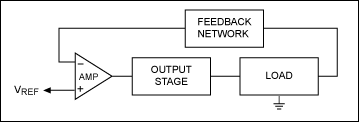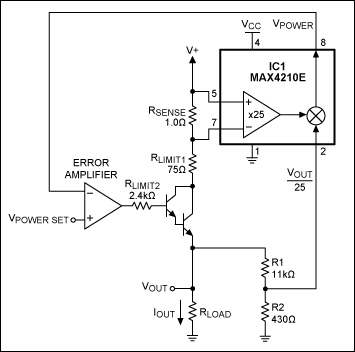# Constant-Power Source

### Abstract

This circuit delivers constant power to a varying load. Using a load-monitoring IC (MAX4210), it senses the load current and load voltage independently, and multiplies the corresponding signals together with an internal analog multiplier. Applying the multiplier output to the inverting input of an error amplifier lets you apply a control voltage to set the constant-power level.

This design idea appeared in the December 15, 2006 issue of Electronic Design magazine.

Supplying a fixed and accurate power level to a load is easy if you know the load impedance, and the impedance doesn't change. Simply apply a constant voltage based on the formula for power in a resistive load: P = V²/R, or V = √PR.

But, how to deliver constant power when the load impedance isn't constant? That problem arises in maintaining a warm LCD display on an outdoor gas pump in cold climates, for instance. As the heating element changes temperature, its resistance changes as well, complicating the delivery of constant power. The variation in resistance is characterized by the temperature coefficient of the heating element. If you apply a constant voltage, power delivered to the load varies inversely with load resistance, as the formula predicts. Variations in applied power (within limits) are acceptable in many applications, but to reduce that variation you must allow the applied voltage to vary with load resistance. The approach makes use of a conventional control loop (Figure 1). For a linear voltage regulator, the error amplifier compares a sample of output voltage to a reference voltage, and forces the output stage to deliver load current at a constant voltage. To maintain constant power instead of constant voltage, however, we must incorporate power into the feedback network. The error amplifier then compares a sample of output power against the reference, and drives the output stage to maintain them equal.The power regulator in Figure 2 delivers a constant power that is linearly proportional to the voltage applied at VPOWER SET. (The ratio is power out/VPOWER SET = 1W/V.) The regulator delivers up to 100mW, driving loads from 10Ω to 500Ω with voltages up to 10V and currents up to 100mA.Figure 3 shows the variation of output power vs. load resistance for several values of the VPOWER SET voltage. It shows that the load power remains fairly constant over a 50:1 variation in load, and for a number of different power settings.The key to this circuit is the high-side power and current monitor IC1 (MAX4210), which includes the circuitry necessary to generate a feedback voltage proportional to the instantaneous load power. It contains a current monitor that measures load current, a buffer that measures load voltage, and an analog multiplier that multiplies the two together, producing an output voltage proportional to load power. The current monitor is a high-side type, in which the sense resistor is connected to the "hot" side of the load instead of the low (ground) side. Thus, high-side monitors avoid adding unwanted resistance in the ground path.

The IC1 version shown (one of several) has a gain of 25 in its current-sense amplifier: for a 1Ω sense resistor, the amplifier output is 25V per ampere of current. The amplifier drives a high-gain Darlington pair to minimize base current, which flows in the load but not through the sense resistor.

The second input for the power measurement is load voltage, which is monitored via a resistor divider with ratio 1:25. That voltage is internally multiplied with the current signal to produce an output proportional to load power:

VPOWER = (VIOUT × 25) × (VOUT/25) = IOUT × VOUT. (1 volt per watt)
By controlling drive to the output stage, the error amplifier forces the IC1 output (proportional to load power) to equal the reference signal at VPOWER SET. The V+ supply voltage (18V) limits the maximum load voltage to approximately 15V. RLIMIT1 and RLIMIT2 limit the load current to approximately 120mA.

This circuit is designed for low-power applications (to 100mW), across a wide range of load impedances. It can easily be scaled, however, to accommodate wide ranges of load current, voltage, and power. To do that, adjust the current-sense resistor for a mid-range value of approximately 50mV (voltage across this resistor must be < 150mV). Similarly, the R1–R2 ratio should be adjusted so the voltage presented to IC1 is roughly 500mV (200mV to 1V yields the accuracy specified for IC1).

Other versions of IC1 incorporate different values of gain, which affects the ratio of power output to the applied VPOWER SET level. Finally, you must consider and modify as required the power-handling capability of RLIMIT1 and the output transistor. This technique is not limited to the linear regulator shown; it applies equally well to many switching regulators, which allow considerably higher output power and higher efficiency.